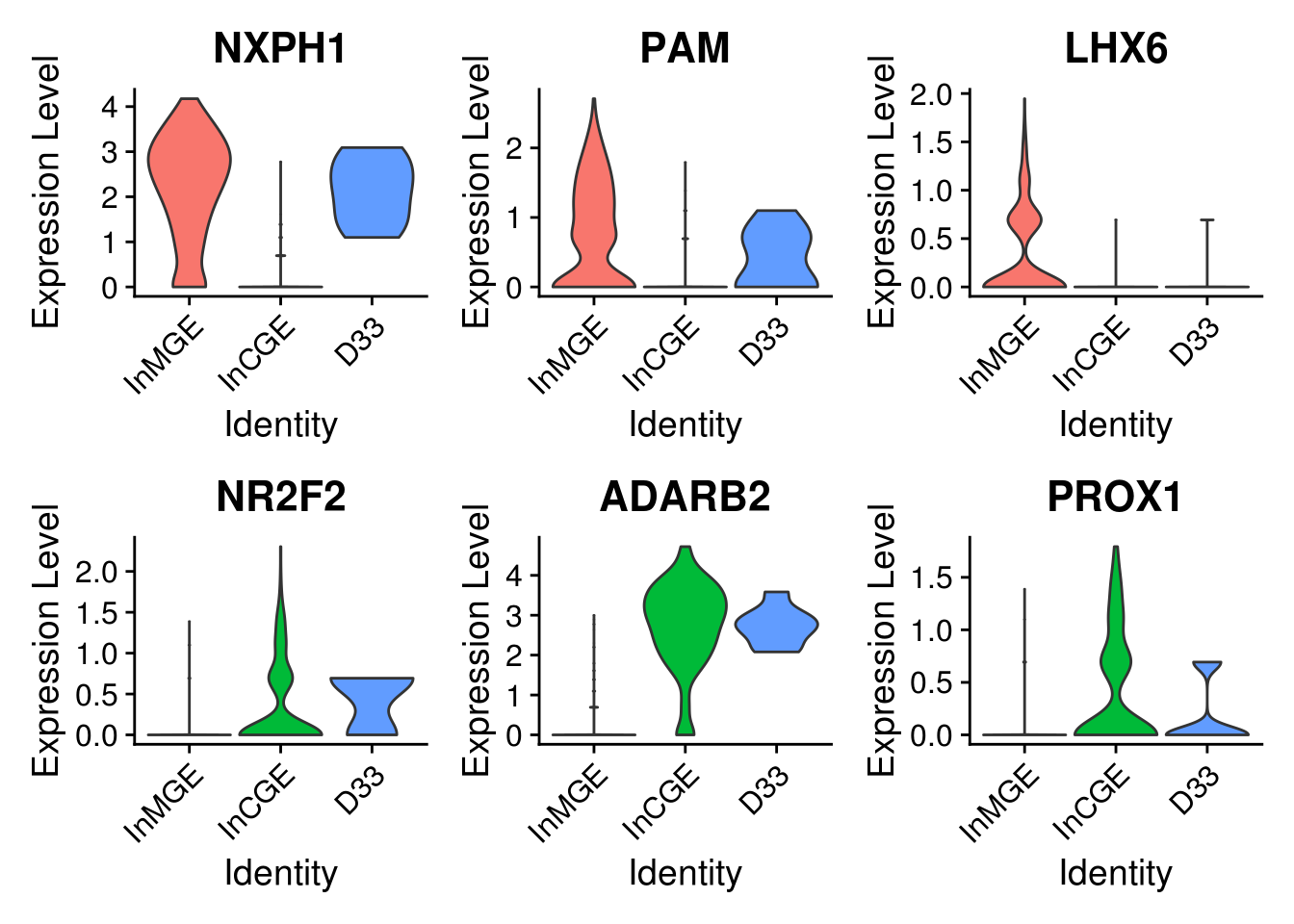# Chapter 4 Scrublet Doublet Validation

``````library(Seurat)
library(tidyverse)
library(magrittr)
library(data.table)``````

## 4.1 Description

• check the doublet prediction from `scrublet` by

• dimension reduction plot

• nUMI distribution

• judge the component for doublet cells by

• DEG heatmap

• canonical gene expression

``````combined <- get(load('data/Demo_CombinedSeurat_SCT_Preprocess.RData'))
Idents(combined) <- "cluster"``````

## 4.3 Validate the doublet prediction

``````# check whether the double cells cluster together
FeaturePlot(combined, features = "DoubletScores", pt.size = 0.01)``````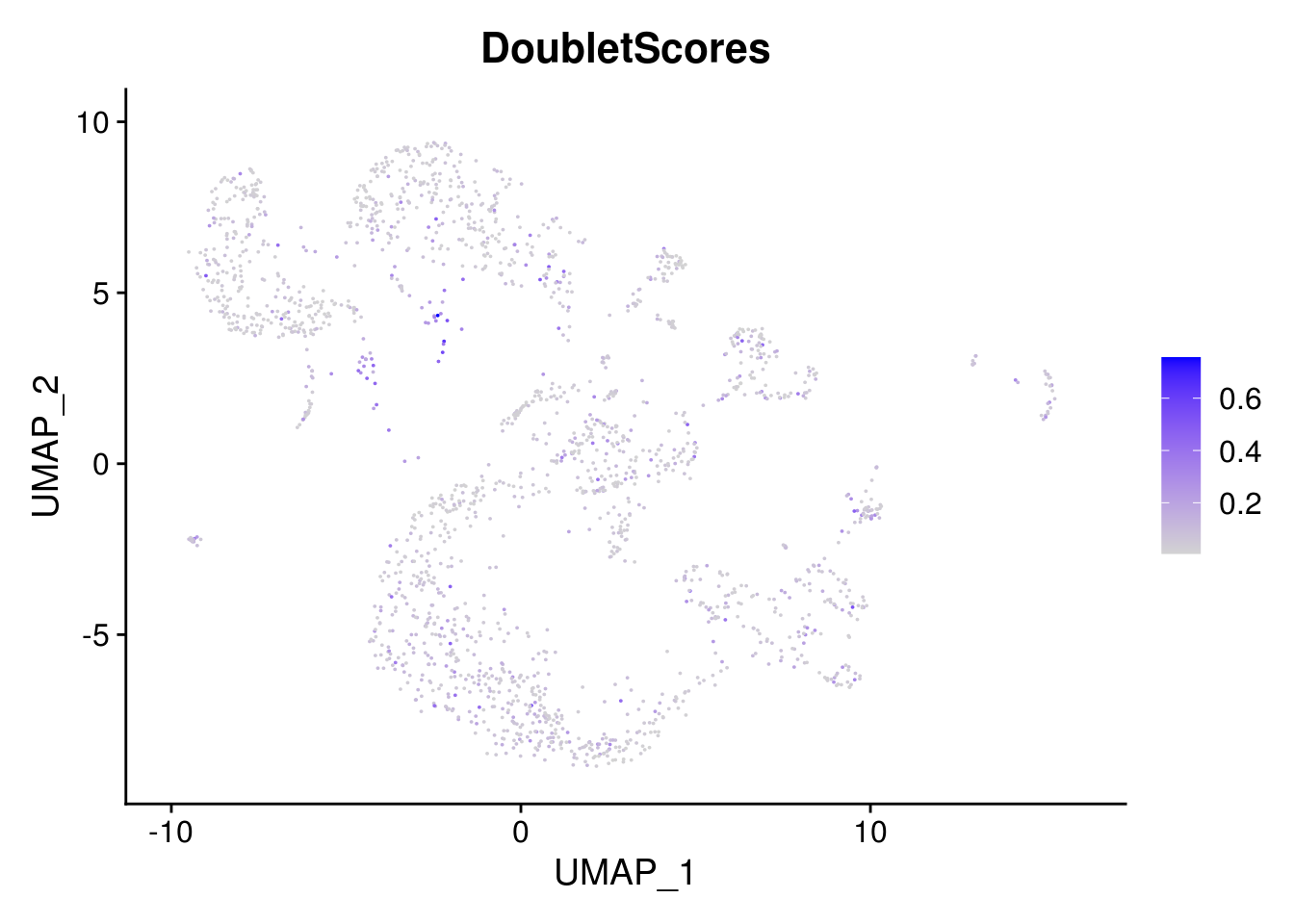``````DimPlot(
combined,
group.by = "DoubletPrediction",
pt.size = 0.01,
cols = c("red", "azure3")
)``````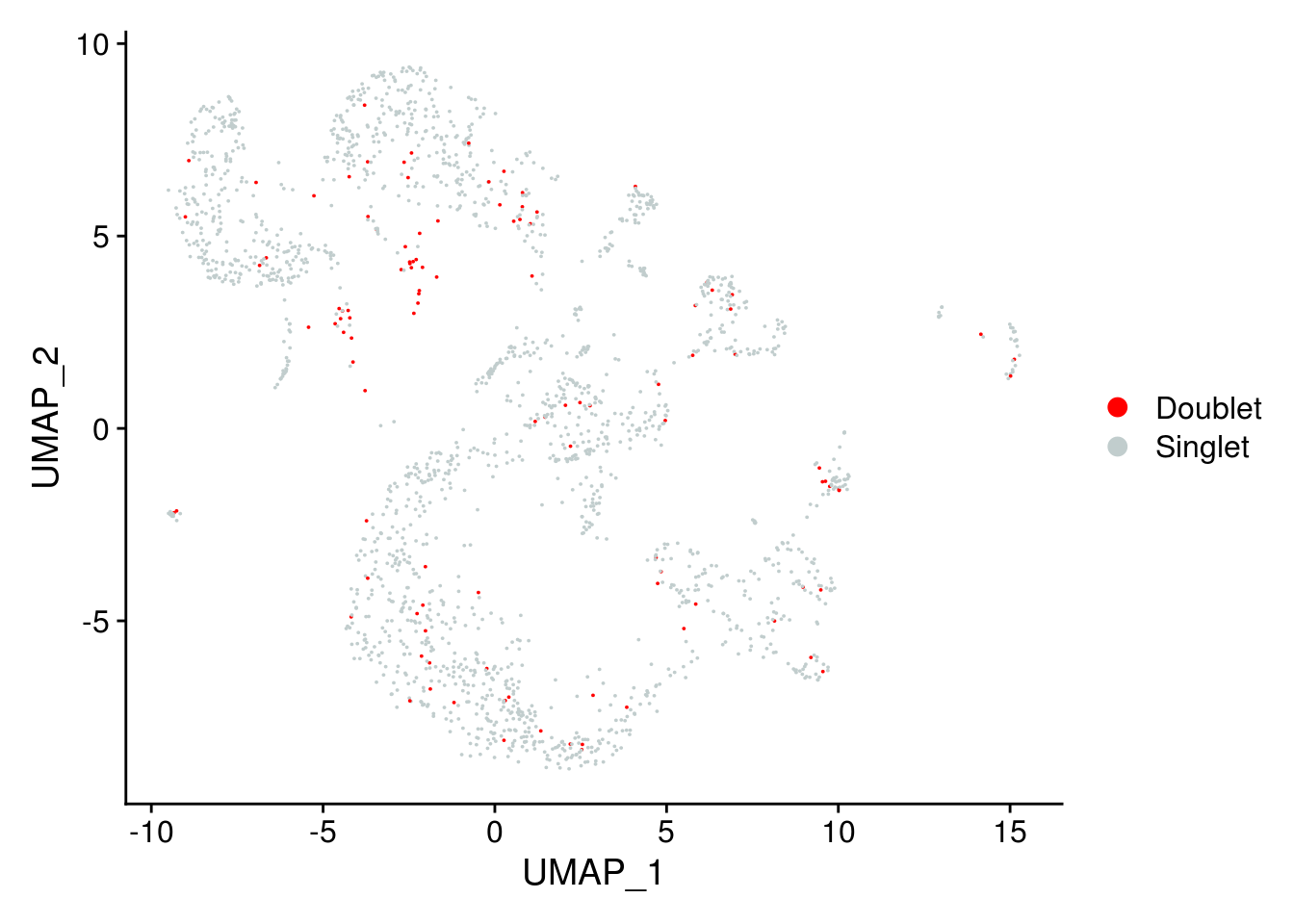``````# check the nUMI for doublet and singlet
VlnPlot(combined,
features = "nCount_RNA",
pt.size = 0,
group.by = "DoubletPrediction") + NoLegend()``````## 4.4 Calculate factions of doublet per cluster

``````df <- data.table(combined@meta.data)
sel.meta <- c("DoubletPrediction", "cluster", "Individual")
df <- df[, sel.meta, with = FALSE]

df[, 2:3] %>% map( ~ {
freq1 <- df[, .N, keyby = .(.x, DoubletPrediction)]
freq1[, total := sum(N), by = .(.x)]
freq1[, ratio := N / total]

linesize = .35
fontsize = 8

ggplot(freq1, aes(fill=DoubletPrediction, y=ratio, x= .x)) +
geom_bar(position="stack", stat="identity")+
scale_fill_manual(values = c("Doublet" = 'red', "Singlet" = "grey")) +
xlab('Clsuter') +
scale_y_continuous(breaks = seq(0,1,0.1), expand = c(0,0), name = 'Percentage')+
theme_bw()+
theme( panel.grid.major.x = element_blank(),
panel.grid.major.y = element_blank(),
panel.grid.minor = element_blank(),
strip.background = element_blank(),panel.border = element_rect(size = linesize),
axis.ticks = element_blank(),
axis.text.x = element_text(size = 5))

})``````
``## \$cluster``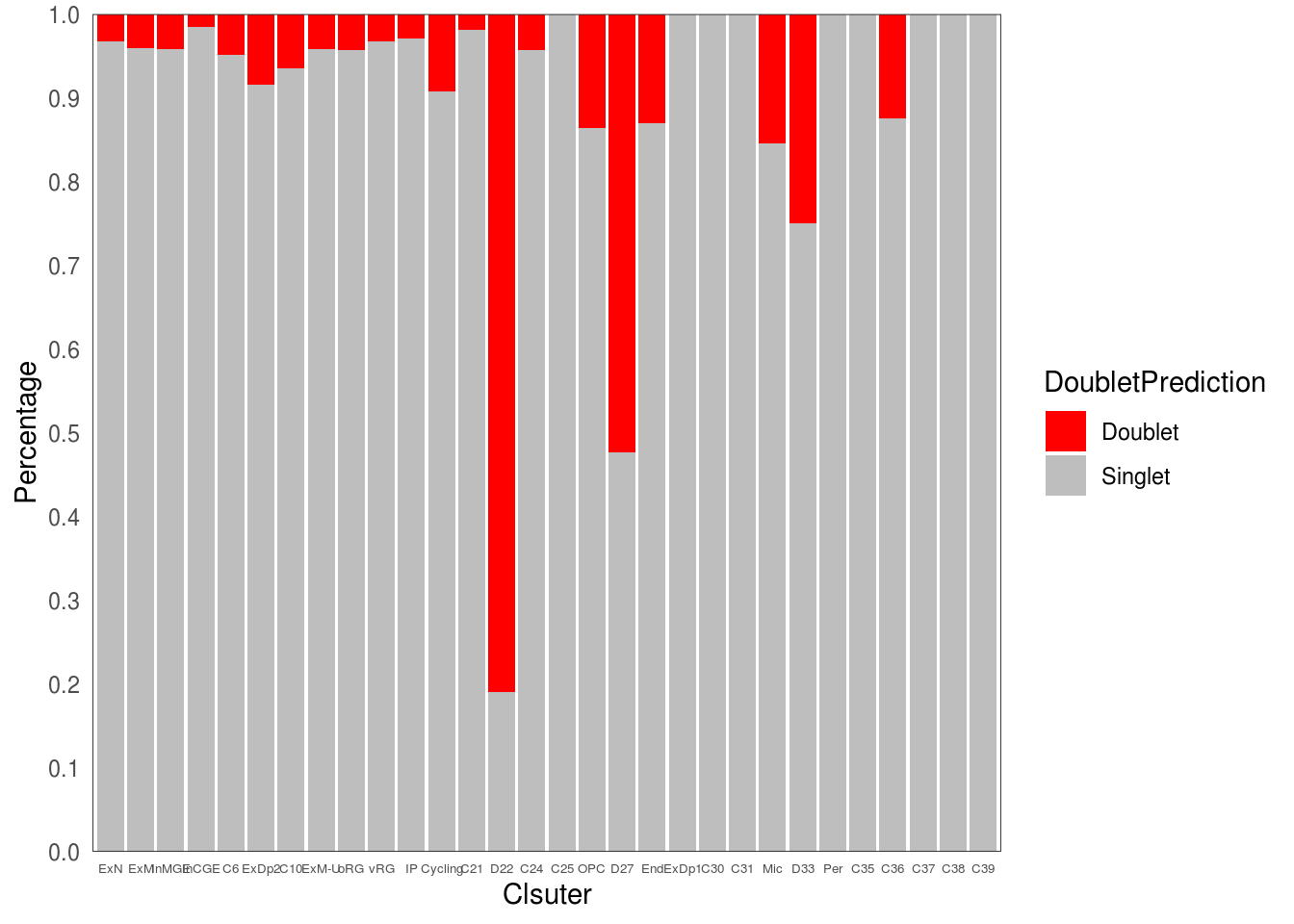``````##
## \$Individual``````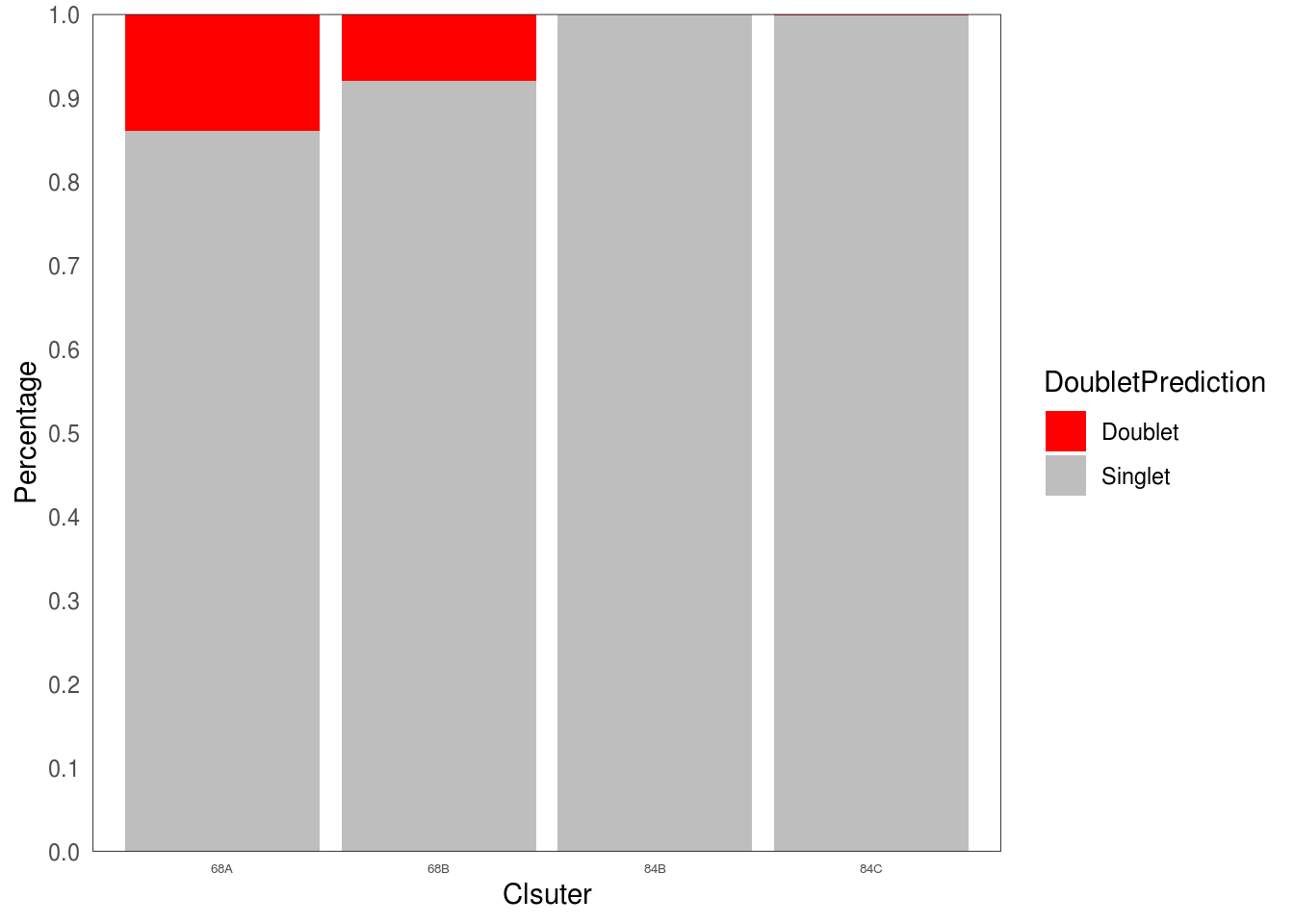## 4.5 Explore the component clusters for doublets by DEG

• get the DEG for inferred source clusters. Here, for C33, InCGE and InMGE
``````# find DEG
cluster.markers <- FindMarkers(combined, ident.1 = c("InMGE"), ident.2 = "InCGE", min.pct = 0.25)

# subset cells of interest
sel.idents <- c("InMGE", "InCGE",  "D33")
combined.small <- subset(combined, cells = WhichCells(combined, idents = sel.idents))

# check the expression for top DEG
#sel.cells <- WhichCells(combined.small, idents = sel.idents, downsample =  355) # for large dataset
DoHeatmap(combined.small, features = rownames(cluster.markers)[1:40], raster = F)``````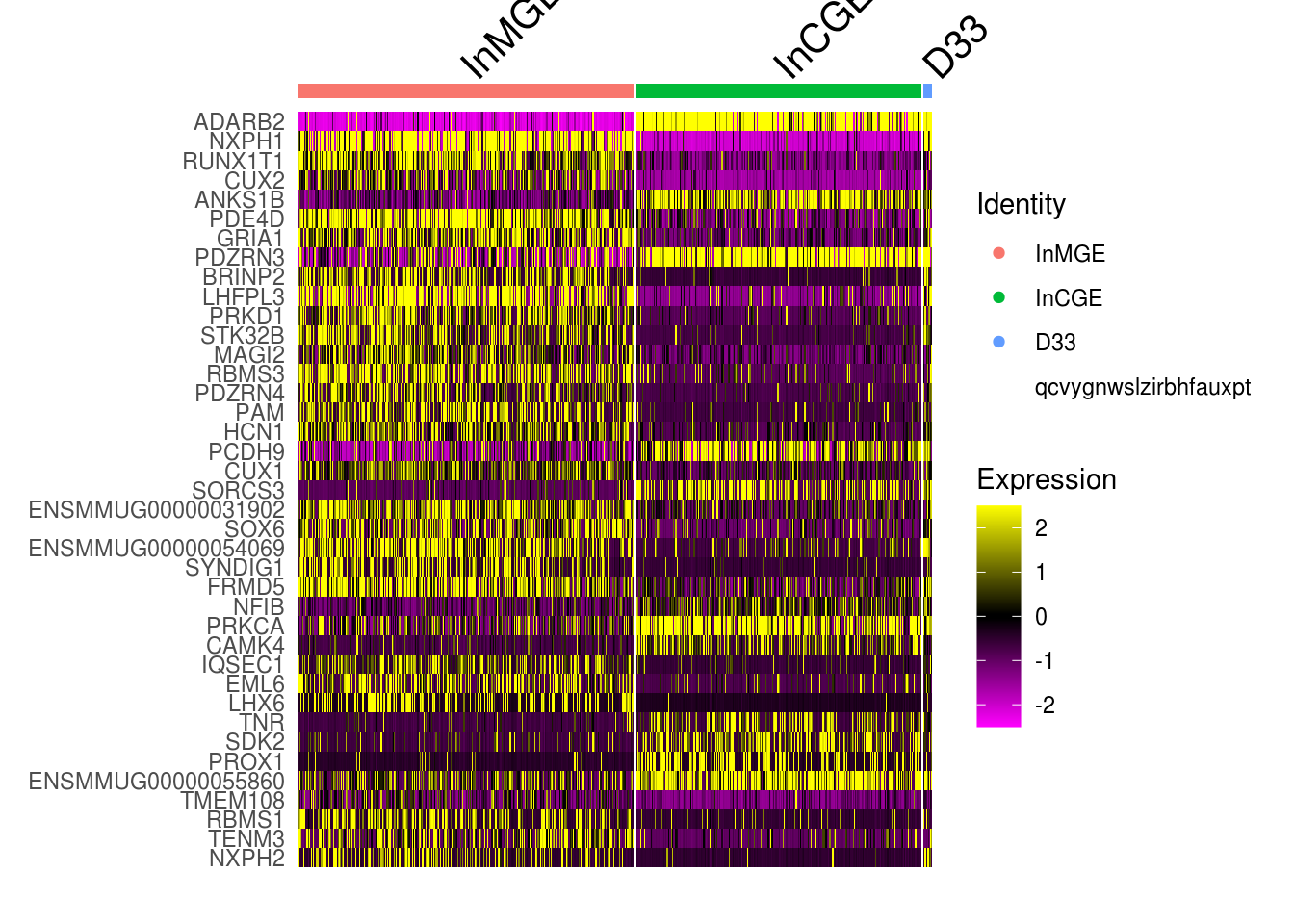## 4.6 Explore the component clusters for doublets by canonical gene

``````sel.feature <- c("NXPH1", "PAM", "LHX6", "NR2F2", "ADARB2",  "PROX1")
FeaturePlot(combined, features = sel.feature,  pt.size = 0.01, ncol = 3)``````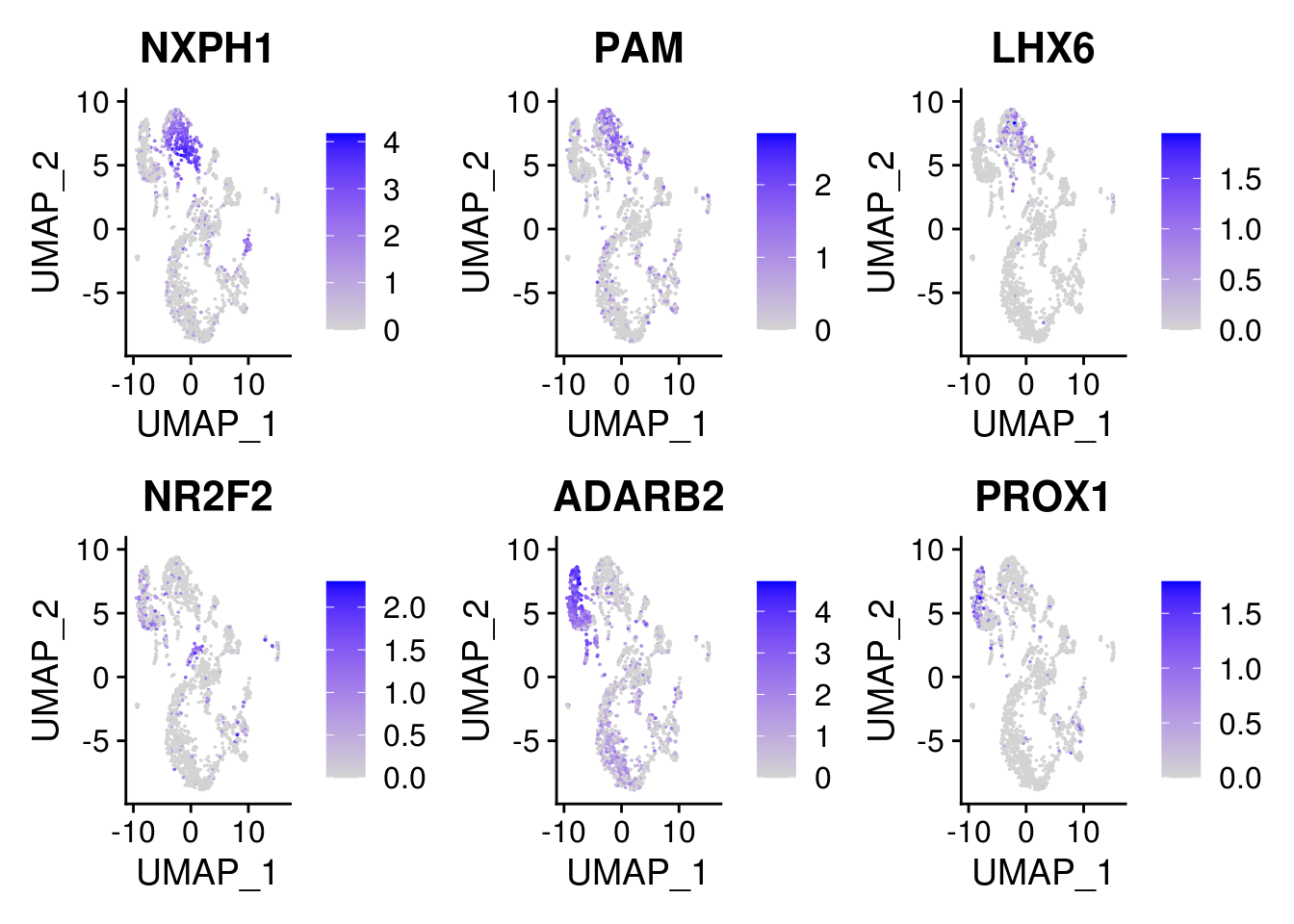``VlnPlot(combined.small, features = sel.feature, pt.size = 0, ncol = 3, idents = sel.idents)``